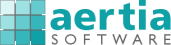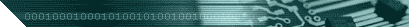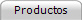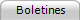Productos: Búsqueda rápida:Por categoríaPor área / sectorPor fabricantePor plataformaDe la A a la ZNovedades Soluciones para: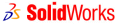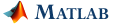Mi perfil:Mi suscripciónMi carrito de compraMis pedidos Sobre Aertia:¿Quiénes somos?Datos de contactoProtección de datosInformación legal

# Time Series Analysis Toolbox for O-Matrix

##### de Harmonic Software

El toolbox STSA (Statistical Time Series Analysis) es una amplia colección de funciones de O-Matrix para realizar el análisis y la visualización de series temporales. Ofrece amplias capacidades para modelos ARMA, ARFIMA, Bayesian, no lineales y espectrales.Descripción | Más información | Demos | Precios | Sectores | PlataformasThe STSA (Statistical Time Series Analysis) Toolbox is an extensive collection of O-Matrix functions for performing time series related analysis and visualization. The STSA toolbox provides extensive capabilities for ARMA and ARFIMA, Bayesian, non-linear and spectral analysis related models. The STSA toolbox aids in the rapid solution of many time series problems, some of which cannot be easily dealt with using a canned program or are not directly available in most analysis software packages. For example, the NONLIN directory provides functions for model selection, estimation and forecasting for the class of functional coefficient autoregressive models: this is a state-of-the-art class of powerful and flexible non-parametric models that can be used in forecasting nonlinear time series. And, in the SPECTRAL directory the user can find functions for simulating, estimating and forecasting long-memory time series, a class of time series that is encountered in such diverse fields as hydrology and finance. The BAYES directory includes functions for Bayesian modeling and forecasting of time series that are not typically available in a commercial statistical package. The Bayesian techniques of this directory offer a greater degree of flexibility than traditional linear models and can handle a large number of forecasting tasks. The ARMA directory provides over 40 functions for analyzing uni- and multidimensional time series via the class of ARMA-type models. A few areas of application of the STSA toolbox include: Forecasting in economics and finance. Sales forecasting and inventory control. Industrial forecasting and process control. Specification of multidimensional models (transfer functions and VARs) and nonlinear models in fields like astronomy, hydrology and operations research. The STSA toolbox can be used by practitioners in a variety of disciplines. It can also be used for classroom instruction, as it allows the instructor to concentrate on the actual application of time series concepts and not on programming. The STSA toolbox offers a complete solution for doing time series analysis: from simulation, to estimation, to residual analysis, to forecasting, complete with many auxiliary statistical functions for descriptive statistics, statistical plots, trend forecasting, time series smoothing and others. The toolbox comes with extensive documentation and numerous examples. STSA Functional Categories Synopsis: ARMA Analysis Univariate Analysis Functions Create a matrix of sequential lags of a time series Estimate and plot the autocovariance function (ACVF), autocorrelation function (ACF) and partial autocorrelation function (PACF) Compute the roots of the characteristic polynomials of an estimate ARIMA/ARFIMA model Compute the coefficients in the infinite AR or MA representation of an ARIMA/ARFIMA model Forecast (predict) future observations of the time series using an estimated model Filter a time series using an estimated model Validate a model using a variety of residual diagnostics Multivariate Analysis Functions Estimate the cross-correlation function (CCF) between pairs of time series Estimate by conditional, nonlinear least squares parameters of a TF model Select the order of a VAR model using two model selection criteria Estimate by conditional linear least squares the parameters of a VAR model Forecast (predict) future observations of the time series using an estimated model Validate a model using a variety of residual diagnostics applied to each individual residual series Bayesian Analysis Simulate a first order polynomial DLM Initialize the Bayesian recursions using arbitrary priors or reference priors Automatically check the observability of a DLM Automatically construct the system and observation matrices for a number of popular DLMs, including DLMs for seasonal time series Fit a variety of DLM models to a time series Perform out of sample forecasting using the fitted DLM Compute interval forecasts and standardized forecast errors Evaluate the forecasting performance of a DLM using the mean squared and mean absolute values of the forecast errors Non-linear Analysis Select the order, delay and threshold values for a TAR model Estimate by conditional, linear least squares the parameters of a selected TAR model Select the order, delay and bandwidth values for a FCAR model Estimate by conditional, linear weighted least squares (WLS) the functional parameters of a selected FCAR model Compute the sequence of one-step ahead forecasts from an estimated FCAR model Spectral Estimate the Fourier transform of a time series either using the discrete Fourier or the fast Fourier method Estimate the periodogram of a time series from the Fourier transform Smooth the periodogram of a time series to obtain its spectrum using a sine taper function Estimate the periodogram of a time series using an AR model approximation Plot the estimated spectrum of a time series System Requirements O-Matrix 5.6 or greater Windows NT, 2000, or XP 1 MB Available Disk SpaceACCIONES » Enviar a un amigo » Imprimir página » Suscribirse a este producto » Ver precios » Ver productos de Harmonic Software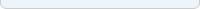© 2004-2005 Aertia Software  •  C/ Sardenya, 229, Sat. 5  •  08013 Barcelona Teléfono: 93 2651320  •  Fax: 93 2652351  •  E-mail: info@aertia.com